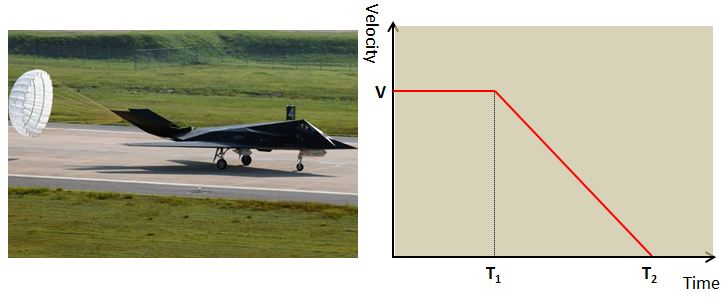# Drag PlaneThe above (left) is an F-$117$ Nighthawk being dragging a parachute to reduce the landing run. Also above (right) is the time-velocity graph of this fighter jet landing after flying with a velocity of $v$. If the velocity before landing doubles, what will be the distance required for the landing? (Assume that the magnitude of air resistance is constant regardless of velocity.)

×